CAT  >  Practice Questions Level 1: Functions - 2

# Practice Questions Level 1: Functions - 2 - Notes | Study Level-wise Practice Questions for CAT Preparation - CAT

 1 Crore+ students have signed up on EduRev. Have you?

This EduRev document offers 20 Multiple Choice Questions (MCQs) from the topic Functions (Level - 1). These questions are of Level - 1 difficulty and will assist you in the preparation of CAT & other MBA exams. You can practice/attempt these CAT Multiple Choice Questions (MCQs) and check the explanations for a better understanding of the topic.

Question for Practice Questions Level 1: Functions - 2
Try yourself:Directions: Answer the question based on the following information.
A function has been defined for a variable x, where domain of F(x) and F1(x) ∈ [-2, 2].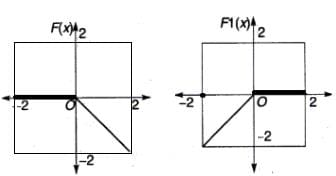Choose the correct option.

Question for Practice Questions Level 1: Functions - 2
Try yourself:If f(x) = x3 + y3, g(x) = xy and h(x) = (x + y), then which of the following is the value of f(x)?

Question for Practice Questions Level 1: Functions - 2
Try yourself:An exponential function is given by f(x) = a3x. If the point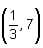lies on the graph of this function, then the value of 'a' is

Question for Practice Questions Level 1: Functions - 2
Try yourself:If f : R - {3} → R - {1} is defined by f(x) =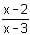, then f is

Question for Practice Questions Level 1: Functions - 2
Try yourself:Which of the following options is necessarily true if f(x) = x3 - 4x + p and f(0) and f(1) have opposite signs?

Question for Practice Questions Level 1: Functions - 2
Try yourself:If f(x) = ax2 + bx + c and c =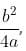then the graph of y = f(x) will certainly

Question for Practice Questions Level 1: Functions - 2
Try yourself:If f(x) = 3x - 5 and f(g(x)) = 2x, then g(x) equals

Question for Practice Questions Level 1: Functions - 2
Try yourself:If f(a, b) = a2 + b3, g(a, b) = a + b, then what is the value of f(3, g(3, 4))?

Question for Practice Questions Level 1: Functions - 2
Try yourself:When the curves y = log10 x and y = x-1 are drawn in the x - y plane, how many times do they intersect for the values x ≥ 1?

Question for Practice Questions Level 1: Functions - 2
Try yourself:Find the minimum value of x2 - 12x + 27.

Question for Practice Questions Level 1: Functions - 2
Try yourself:A balloon, which always remains spherical, has a variable radius. Find the rate at which its volume is increasing with respect to its radius, when the radius is 7 cm.

Question for Practice Questions Level 1: Functions - 2
Try yourself:Find the two numbers such that their sum is 12 and the sum of their squares is minimum.

Question for Practice Questions Level 1: Functions - 2
Try yourself:The sum of two numbers is 2k. The numbers such that the sum of their squares is minimum, will be

Question for Practice Questions Level 1: Functions - 2
Try yourself:Refer to the following data to answer the question given below:
R(x, y) =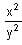and sq(x, y) =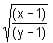rem (x, y) = remainder when x is divided by y
q(x, y) = quotient when x is divided by y
sq(5, 10) is equal to

Question for Practice Questions Level 1: Functions - 2
Try yourself:If f(x) =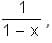then f[f[f(x)]] equals

Question for Practice Questions Level 1: Functions - 2
Try yourself:Radius of a circle is increasing uniformly at the rate of 3 cm/sec. The rate of increase in area, when radius is 10 cm will be

Question for Practice Questions Level 1: Functions - 2
Try yourself:Differentiate 3y3 + 4y2 - 6y + 7 w.r.t. y.

Question for Practice Questions Level 1: Functions - 2
Try yourself:If f(x) is an even function, then the graph y = f(x) will be symmetrical about

Question for Practice Questions Level 1: Functions - 2
Try yourself:If f (x) is an odd function, then the graph y = f(x) will be symmetrical about

Question for Practice Questions Level 1: Functions - 2
Try yourself:Find the minimum value of the function f (x) = log2 (x2 – 2x + 5).

The document Practice Questions Level 1: Functions - 2 - Notes | Study Level-wise Practice Questions for CAT Preparation - CAT is a part of the CAT Course Level-wise Practice Questions for CAT Preparation.
All you need of CAT at this link: CAT

## Level-wise Practice Questions for CAT Preparation

277 docs
 Use Code STAYHOME200 and get INR 200 additional OFF

## Level-wise Practice Questions for CAT Preparation

277 docs

### How to Prepare for CAT

Read our guide to prepare for CAT which is created by Toppers & the best Teachers

Track your progress, build streaks, highlight & save important lessons and more!

,

,

,

,

,

,

,

,

,

,

,

,

,

,

,

,

,

,

,

,

,

;